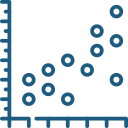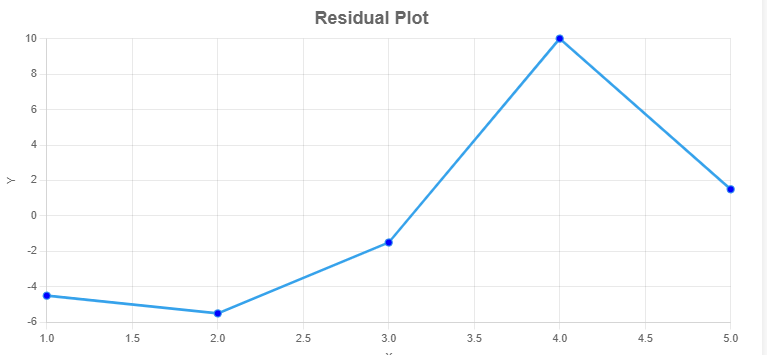Uh Oh! It seems you’re using an Ad blocker!

We always struggled to serve you with the best online calculations, thus, there's a humble request to either disable the AD blocker or go with premium plans to use the AD-Free version for calculators.

Or# Residual Plot Calculator

Enter the dependent and independent variable in the tool to find the residual plot

Independent variable X data, separated with comma (,):

Dependent variable Y data, separated with comma (,):

Table of Content

Get the Widget!

Add this calculator to your site and lets users to perform easy calculations.

Feedback

How easy was it to use our calculator? Did you face any problem, tell us!

The residual plot calculator gives you the graphical representation of the observed and the residual points of statistical data with the proper steps shown.

The residual scatter plot provides a clear picture of the difference between the predicted and the actual values in the regression analysis.

## What is the Residual Plot?

The residual scatter plot is the vertical distance data set point and a regression line. The residual plot checks the linear regression model. It quickly assesses how much the observed values violated the predicted values.

The Residual plot maker values usually drift from the predicted observation as in the Sum of Square Error(SSE). The residual scatterplot clarifies the deviation of the difference between the observed values and predicted values.

### Types of the Residual Scatterplot:

There are different types of residual plots:

• Residuals versus observation values
• Normality plot of residuals
• Residuals versus predicted value plot

## How to Make Residual Plot?

To make a residual plot online, you require the predicted values and residual values:

### The Predicted Values:

After finding the regression equation, we can gather the predicted values by inserting the independent variable in the regression equation.

$$hat{Y} = 6.25 + 0.25X$$

### The Residual Values:

The predicted and residual values are given in the table below:

 Obs. X Y Predicted Values Residuals value=(Y-P.V) 1 1 2 6.25 + 0.25 × 1 = 6.5 2 – 6.5 = -4.5 2 13 4 6.25 + 0.25 × 13 = 9.5 4 – 9.5 = -5.5 3 5 6 6.25 + 0.25 × 5 = 7.5 6 – 7.5 = -1.5 4 7 18 6.25 + 0.25 × 7 = 8 18 – 8 = 10 5 9 10 6.25 + 0.25 × 9 = 8.5 10 – 8.5 = 1.5

### The Residual Plot:

The residual plot is the result produced by the residuals versus the observation number. Try to make a residual plot online to identify the difference between the predicted values and observed values.A residual plot represents the difference between the actual response and the observed response of the statistical values. The residual plot grapher represents the pattern of the residual point relative to the observed values and describes the standard deviation.

## Working of the Residual Plot Calculator:

The residual plot calculator draws the graph between the observed values and actual values by the following procedure:

Input:

• Enter the dependent(X) and independent variable (Y)
• Tap Calculate

Output:

• The residual plot
• Step-by-step calculation

## FAQs:

### What is Meant by Heteroscedasticity?

Heteroskedasticity refers to situations where the variance of the residuals shows too much difference between the actual and observed values. The residual graph calculator makes sure the residual points are showing Heteroskedasticity or not.

### What Does a Curved Residual Plot Mean?

If the residual points in the residual scatter plot appear in the curved pattern. It means the regression model you have specified is not correct.

## References:

From the source of Analyse-it.com: Residual plot, Why draw residual plot?

From the source of Statisticshowto.com: Definition and Examples